## Pages

### New GCSE Support

The main additions to the syllabus are listed below (this list is not exhaustive). Information and resources are provided for each new topic. This page is work in progress and will be updated regularly. Please email any additional resources to resourceaholic@gmail.com.

Functions"Interpret simple expressions as functions with inputs and outputs; interpret the reverse process as the ‘inverse function’; interpret the succession of two functions as a ‘composite function'"

My blog post 'New GCSE: Functions' describes the specification, resources and teaching ideas.

Functions Subject Knowledge Audit by @MrDraperMaths

These resources (including sources) are listed in my algebra library.Quadratic Graphs
"Identify and interpret roots, intercepts, turning points of quadratic functions graphically; deduce roots algebraically and turning points by completing the square"

My blog post describes methods for finding the vertex of a quadratic function.

Quadratic Functions Subject Knowledge Audit by @DrBennison

Resources:
Quadratic pointsQuadratic discs / Quadratic Functions / Sketching Quadratics / Odd One Out / Completing the square activity / Forming Quadratics / Quadratics card sort / Completing the square to sketch / Quadratic matching / Translating y = x squared / Quadratics from roots, vertex and y-intercept / Which graph? / Graph MatchGraphs of Quadratic Equations / Representing Quadratic Functions Graphically /  Geogebra file / Edexcel A11 Quadratic functions - roots, intercepts, turning points / Edexcel A11 Deduce turning points by completing the square
These resources (including sources) are listed in my algebra library

These resources (including sources) are listed in my algebra library.Iteration
"Find approximate solutions to equations numerically using iteration"

Iterative Methods Subject Knowledge Audit by @DrBennison

Blog post by Colleen Young - GCSE New Content – Iterative Methods for Numerical Solution of Equations

Blog post by Peter Mattock - Iteration and the new GCSE

Iteration Worked Solution Card Match - SiouxzieD on TES
Bridging Units: Resource Pocket 4 - Iterative methods for solving equations numerically - AQA

Solving cubic equations iteratively - Don Steward
Destination Douze and Iteration - Don Steward

A20 Iterations - Edexcel

Iteration to solve equations - toticity.co.uk

Inequalities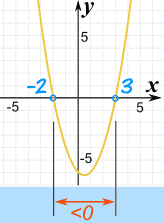"Solve linear inequalities in one or two variable(s), and quadratic inequalities in one variable; represent the solution set on a number line, using set notation and on a graph".

My blog post about new content and methods

Resources:

These resources (including sources) are listed in my algebra library.

ICT tools:
Geogebra 1Geogebra 2 / Desmos / echalk

Sequences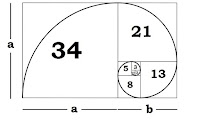"Recognise and use sequences of triangular, square and cube numbers, simple arithmetic progressions, Fibonacci type sequences, quadratic sequences, and simple geometric progressions (rn where n is an integer, and r is a rational number > 0 or a surd) and other sequences"

My blog post New GCSE: Sequences describes the new content, provides example questions and features links to resources.

Resources:
These resources (including sources) are listed in my algebra library.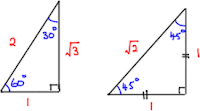Exact Trig Values
"Know the exact values of sinθ and cosθ for θ = 0o, 30o, 45o, 60o and 90o; know the exact value of tanθ for θ = 0o, 30o, 45o and 60o"

Exact Trig Ratios Subject Knowledge Audit by @DrBennison

Resources:
Exact trigonometric values / Exact trig values worksheet / Exact trig values codebreaker /
Finding and applying the 3 trigonometric ratios

These resources (including sources) are listed in my shape library.

Frequency Trees
"Record describe and analyse the frequency of outcomes of probability experiments using tables and frequency trees"

Blog post: Using expected frequencies when teaching probability

Resources:
Frequency Trees / Frequency tree - explanation and explorationPrize Giving / Learn Homework / Frequency Trees

These resources (including sources) are listed in my data library.

Error Intervals"Use inequality notation to write down an error interval for a number or measurement rounded or truncated to a given degree of accuracy."

Blog post: GCSE 9-1 New content – Error Intervals from Just Maths

Resources:
Bounds and Error Intervals / Errors in Truncation / Edexcel N15 Bounds / TruncationSets and Venns
"Enumerate sets and combinations of sets systematically, using tables, grids, Venn diagrams and tree diagrams."

These resources (including sources) are listed in my data library.

Multiplicative Counting and Systematic Listing
"Apply systematic listing strategies including use of the product rule for counting"

Systematic listing and counting strategies - resources available with MathsPad subscription

Three pens - Just Maths

Blog post: Multiplicative counting - the different types from @MrMattock

Blog post & resource list: Systematic Listing Strategies from @ColleenYoung

Invariance"Describe the changes and invariance achieved by combinations of rotations, reflections and translations"

Invariance activity sheet

More resources coming soon!

Shortest distance of a point from a line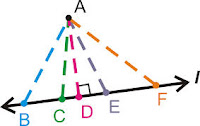"Know that the perpendicular distance from a point to a line is the shortest distance to the line"

Geogebra file 1
Geogebra file 2

Resources coming soon!"Interpret the gradient at a point on a curve as the instantaneous rate of change; apply the concepts of average and instantaneous rate of change (gradients of chords and tangents) in numerical, algebraic and graphical contexts"

Tangents and Areas Subject Knowledge Audit by @DrBennison

My blog post about content, methods and resources.

Resources:

These resources (including sources) are listed in my algebra library.

Area under a graph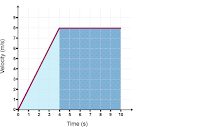"Calculate or estimate gradients of graphs and areas under graphs (including quadratic and other non-linear graphs), and interpret results in cases such as distance-time graphs, velocity-time graphs and graphs in financial contexts"

Tangents and Areas Subject Knowledge Audit by @DrBennison

My blog post about content, methods and resources.

Resources:

These resources (including sources) are listed in my algebra library.

Expanding cubics"Simplify and manipulate algebraic expressions (including those involving surds and algebraic fractions) by expanding products of two or more binomials"

Blog Post: GCSE 9 -1 New content – Two or more binomials from Just Maths

Resources:
Expanding PolynomialsGrid method polynomial expansions / Edexcel A4 Expand products of two or more binomials / Geogebra file / Expanding binomials – Squares / Triple Brackets – Boarding Card

These resources (including sources) are listed in my algebra library.

"Set up, solve and interpret the answers in growth and decay problems, including compound interest and work with general iterative processes"

Resources:
Exam questions / Exponential growth and decay / OCR check-in test / AQA topic test / AQA Bridging Unit growth and decay / Exponential functions / Compound interest / Growth and Decay / Exponential growth and decay / Compound percentages activities

These resources (including sources) are listed in my number library.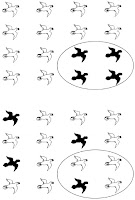Capture-Recapture (Edexcel)
"Infer properties of populations or distributions from a sample, while knowing the limitations of sampling. To include the calculation of summary statistics from a sample, knowing that these are estimates for the population. At Higher tier, to include the Peterson capture–recapture method"

Blog post: New GCSE: Capture-Recapture

These resources (including sources) are listed in my data library.

Time Series"Interpret and construct tables, charts and diagrams, including ... tables and line graphs for time series data and know their appropriate use."

Resources:
Time Series Graphs / Data Analysis and Interpretation / Time Series Graphs / Time Series Chapter

These resources (including sources) are listed in my data library.

Scatter Graphs"Use and interpret scatter graphs of bivariate data; recognise correlation and know that it does not indicate causation; draw estimated lines of best fit; make predictions; interpolate and extrapolate apparent trends whilst knowing the dangers of so doing."

My blog post about scatter graphs

Resources:
Scatter Graphs True or FalseCorrelation vs Causation Worksheet / Correlation vs Causation Activities / Causation Examples / Correlation and cause slides

More resources coming soon!

These resources (including sources) are listed in my data library.

My posts:
Five things you might not know about the new GCSE content #1

My post on Resources for New GCSE Topics

GCSE New Content by Colleen Young

Probability by Colleen Young

Content Changes by Just Maths

Planning for GCSE 2015 by Miss Norledge

Free resources from OCR

Bridging the Gap Resources from AQA

Exam Questions by Topic (Higher) - Just Maths

Exam Questions by Topic (Foundation) - Just Maths

Exam Questions by Topic (Both tiers) - Just Maths

Exam Questions - Maths Genie

Revision booklets - pixi_17 on TES

9 - 1 GCSE Help Book - m4ths.com

1.Thank you for this post... our maths department need to prepare a resource to use for the new style GCSE & present at tonight's meeting. It has been suggested that we start by looking here - what a compliment to you that a whole department are using your blog!

1.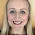That's fab - thank you! Great idea for a department meeting too.

2.Hi, thank you for these resources they have been amazing for putting our SOL together and providing guidance to the department. Do you have any clarification on what is meant by "vertical line charts" in statistics point 2? Is this just a line graph?

1.Thanks! I'm glad it's useful. I believe a 'vertical line chart' is just a bar chart with lines instead of full bars (ie height of each vertical line represents frequency). So different to a line graph, which is a time series graph (ie plot points and join with straight lines).

3.Thank you so much for sharing these resources, they have undoubtedly saved us a lot of time, and I am extremely grateful.

1.4.5.1.